Practice questions available in McGraw Hill Math Grade 6 Answer Key PDF Lesson 11.1 Adding Decimals will engage students and is a great way of informal assessment.

Question 1.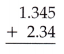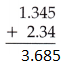The sum of two decimal numbers 1.345 and 2.34 is 3.685

Question 2.The sum of two decimal numbers 7.772 and 3.333 is 11.105

Question 3.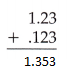The sum of two decimal numbers 1.23 and 0.123 is 1.353

Question 4.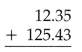The sum of two decimal numbers 12.35 and 125.43 is 137.78

Question 5.The sum of two decimal numbers 4.4224 and 44.2442 is 48.6666

Question 6.The sum of two decimal numbers 53.111 and 0.3341 is 53.4451

Question 7.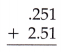The sum of two decimal numbers 0.251 and 2.51 is 2.761

Question 8.The sum of two decimal numbers 10.101 and 0.990 is 11.091

Question 9.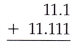The sum of two decimal numbers 11.1 and 11.111 is 22.211

Question 10.The sum of two decimal numbers 113.31 and 31.113 is 144.423

Question 11.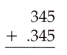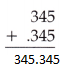The sum of two decimal numbers 345 and 0.345 is 345.345

Question 12.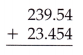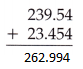The sum of two decimal numbers 239.54 and 23.454 is 262.994

Question 13.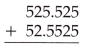The sum of two decimal numbers is 525.525 and 52.5525 is 578.0775

Question 14.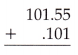The sum of two decimal numbers is 101.55 and 0.101 is 101.651

Question 15.
Hailey is on the school track team. Her event is the triple jump. In her last meet the “hop” portion of her jump was 12.256 feet, the “skip” 11.114 feet, and the “jump” portion was 13.4455 feet. How far did she jump in total?
Given,
Hailey is on the school track team. Her event is the triple jump.
In her last meet the “hop” portion of her jump was 12.256 feet, the “skip” 11.114 feet, and the “jump” portion was 13.4455 feet.
12.256 + 11.114 + 13.4455 = 36.8155 feet
Thus she jumps 36.8155 feet in total.

Question 16.
During a flight to the International Space Station, the space shuttle pilot had to conduct a mid-course correction by firing the shuttle’s rockets three times lasting 12.354 seconds, 11.4538 seconds, and 9.7392 seconds. What is the total time the rockets were fired?